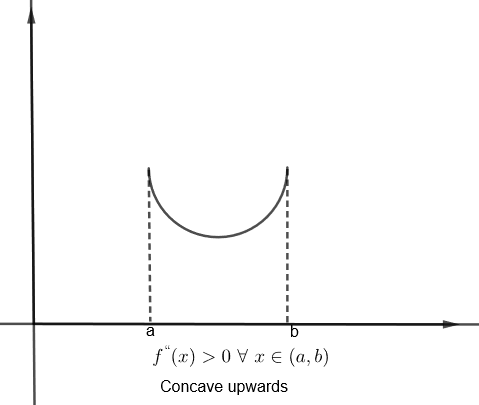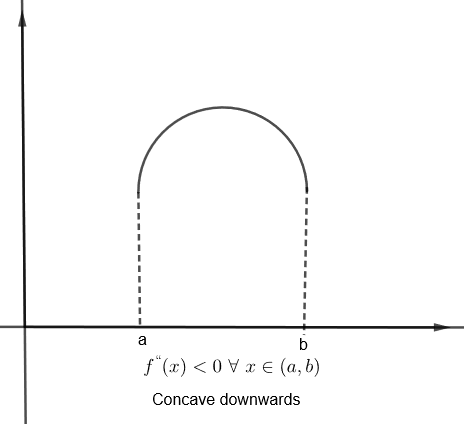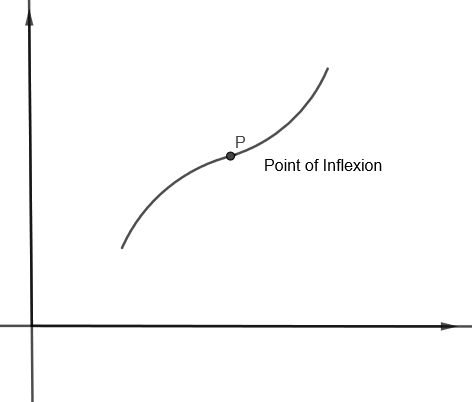Courses
Courses for Kids
Free study material
Free LIVE classes
MoreLIVE
Join Vedantu’s FREE Mastercalss

# Find the intervals of concavity and the points of inflexion of the function $f\left( x \right)={{\left( x-1 \right)}^{\dfrac{1}{3}}}$Verified
362.4k+ views
Hint: Double differentiate the given f(x) and find whether it is positive or negative. When f''(x) > 0, then the graph of f (x) is concave upwards and when f''(x )< 0, then the graph of f (x) is concave downwards. Also we will get the inflexion point at x where f'' (x) = 0 or does not exist.

Here we have to find the intervals of concavity and points of inflexion of function
$f\left( x \right)={{\left( x-1 \right)}^{\dfrac{1}{3}}}$
We know that if double derivative of function which is ${{f}^{''}}\left( x \right)>0\text{ }\forall \text{ }x\in \left( a,b \right)$, then the graph of f (x) is concave upwards in (a, b).
Similarly, if ${{f}^{''}}\left( x \right)<0\text{ }\forall \text{ }x\in \left( a,b \right)$, then the graph of f (x) is concave downwards in (a, b).The point at which concavity of graph changes, i.e. $\dfrac{{{d}^{2}}x}{d{{y}^{2}}}=0$ or does not exist is the point of inflexion.
The sign of $\dfrac{{{d}^{2}}x}{d{{y}^{2}}}$ changes about the point of inflexion.Now, we can take
$f\left( x \right)={{\left( x-1 \right)}^{\dfrac{1}{3}}}$
We know that,
$\dfrac{d}{dx}\left( {{x}^{n}} \right)=n{{x}^{n-1}}$
Now, we will differentiate f (x). We get,
${{f}^{'}}\left( x \right)=\dfrac{1}{3}{{\left( x-1 \right)}^{\dfrac{1}{3}-1}}$
${{f}^{'}}\left( x \right)=\dfrac{1}{3}{{\left( x-1 \right)}^{\dfrac{-2}{3}}}$
Now, again we will differentiate the above equation. We get,
${{f}^{''}}\left( x \right)=\dfrac{1}{3}\left( \dfrac{-2}{3} \right){{\left( x-1 \right)}^{\dfrac{-2}{3}-1}}$
${{f}^{''}}\left( x \right)=\dfrac{-2}{9}{{\left( x-1 \right)}^{\dfrac{-5}{3}}}$
Or, ${{f}^{''}}\left( x \right)=\dfrac{-2}{9{{\left( x-1 \right)}^{\dfrac{5}{3}}}}$
We know that the point of inflexion is a point where ${{f}^{''}}\left( x \right)=0$ or does not exist. It changes its sign.
As we can see that ${{f}^{''}}\left( x \right)$ cannot be zero but ${{f}^{''}}\left( x \right)$ will not exist at x = 1 as (x – 1) which is in the denominator will become zero at x = 1.
Now, for x > 1, (x – 1) would be greater than zero. So,
${{\left( x-1 \right)}^{\dfrac{5}{3}}}>0$
Therefore,
${{f}^{''}}\left( x \right)=\dfrac{-2}{9{{\left( x-1 \right)}^{\dfrac{5}{3}}}}<0$
As for any value of x, say x = 2 in this case, we would get the value of ${{f}^{''}}\left( x \right)\text{ as }\dfrac{-2}{9}$.
Now, for x < 1, (x – 1) would be less than zero. So,
${{\left( x-1 \right)}^{\dfrac{5}{3}}}<0$
Therefore,
${{f}^{''}}\left( x \right)=\dfrac{-2}{9{{\left( x-1 \right)}^{\dfrac{5}{3}}}}>0$
As for any value of x, say x = 0 in this case, we would get the value of ${{f}^{''}}\left( x \right)\text{ as }\dfrac{2}{9}$.
Therefore, as f”(x) does not exist at x = 1, and f”(x) is positive for x < 1 and negative for x > 1, that is f (x) changes its sign about the point x = 1. Therefore, x = 1 is inflexion point.
As we have proved that f”(x) > 0 for x < 1
Therefore, f(x) is concave upwards for $\left( -\infty ,1 \right)$
As we have proved that f”(x) < 0 for x > 1
Therefore, f(x) is concave downwards for $\left( 1,\infty \right)$

Note: Students should always check if the function is changing its sign at an inflexion point and not only rely upon $\dfrac{{{d}^{2}}y}{d{{x}^{2}}}=0$ or does not exist for finding the inflexion point.
Last updated date: 27th Sep 2023
Total views: 362.4k
Views today: 4.62k